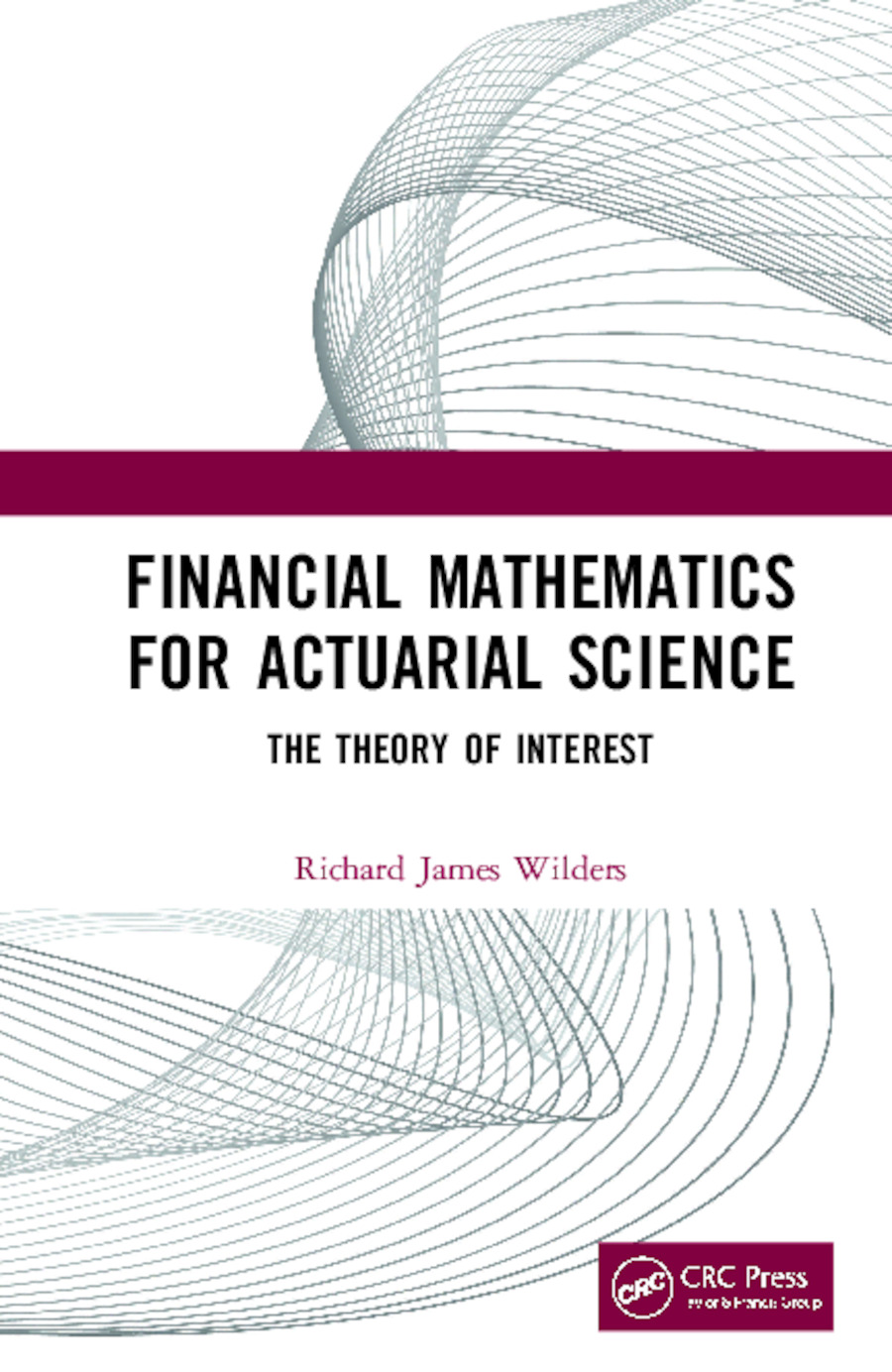# Financial Mathematics For Actuarial Science

## The Theory of Interest, 1st Edition

CRC Press

396 pages | 36 B/W Illus.

Hardback: 9780367253080
pub: 2020-02-18
Available for pre-order. Item will ship after 18th February 2020
\$120.00
x

FREE Standard Shipping!

### Description

Financial Mathematics for Actuarial Science: The Theory of Interest is concerned with the measurement of interest and the various ways interest affects what is often called the time value of money (TVM). Interest is most simply defined as the compensation that a borrower pays to a lender for the use of capital. The goal of this book is to provide the mathematical understandings of interest and the time value of money needed to succeed on the actuarial examination covering interest theory

Key Features

• Helps prepare students for the SOA Financial Mathematics Exam
• Provides mathematical understanding of interest and the time value of money needed to succeed in the actuarial examination covering interest theory
• Contains many worked examples, exercises and solutions for practice
• Provides training in the use of calculators for solving problems
• A complete solutions manual is available to faculty adopters online

1. Overview and Mathematical Prerequisites. 2. Measuring Interest. 3. Solving Problems in Interest. 4. Annuities. 5. Amortization Schedules and Sinking Funds. 6. Yield Rates. 7. Bonds. 8. Exact Asset Matching and Swaps. 9. Interest Rate Sensitivity. 10. Determinants of Interest Rates.